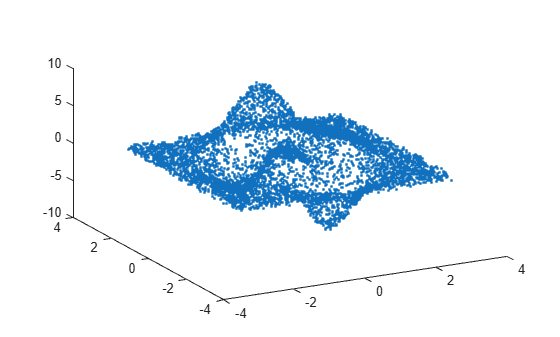# Average Similar Data Points Using a Tolerance

This example shows how to use `uniquetol` to find the average `z`-coordinate of 3-D points that have similar (within tolerance) `x` and `y` coordinates.

Use random points picked from the `peaks` function in the domain $\left[-3,3\right]×\left[-3,3\right]$ as the data set. Add a small amount of noise to the data.

```xy = rand(10000,2)*6-3; z = peaks(xy(:,1),xy(:,2)) + 0.5-rand(10000,1); A = [xy z]; plot3(A(:,1), A(:,2), A(:,3), '.') view(-28,32)```Find points that have similar `x` and `y` coordinates using `uniquetol` with these options:

• Specify `ByRows` as `true`, since the rows of `A` contain the point coordinates.

• Specify `OutputAllIndices` as `true` to return the indices for all points that are within tolerance of each other.

• Specify `DataScale` as `[1 1 Inf]` to use an absolute tolerance for the `x` and `y` coordinates, while ignoring the `z`-coordinate.

```DS = [1 1 Inf]; [C,ia] = uniquetol(A, 0.3, 'ByRows', true, ... 'OutputAllIndices', true, 'DataScale', DS);```

Average each group of points that are within tolerance (including the `z`-coordinates), producing a reduced data set that still holds the general shape of the original data.

```for k = 1:length(ia) aveA(k,:) = mean(A(ia{k},:),1); end```

Plot the resulting averaged-out points on top of the original data.

```hold on plot3(aveA(:,1), aveA(:,2), aveA(:,3), '.r', 'MarkerSize', 15)```